2022: SklogWiki celebrates 15 years on-line

# Ising model

(diff) ← Older revision | Latest revision (diff) | Newer revision → (diff)

The Ising model  (also known as the Lenz-Ising model) is commonly defined over an ordered lattice. Each site of the lattice can adopt two states,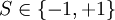$S \in \{-1, +1 \}$. Note that sometimes these states are referred to as spins and the values are referred to as down and up respectively. The energy of the system is the sum of pair interactions between nearest neighbors.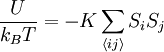$\frac{U}{k_B T} = - K \sum_{\langle ij \rangle} S_i S_j$

where$k_B$ is the Boltzmann constant,$T$ is the temperature,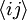$\langle ij \rangle$ indicates that the sum is performed over nearest neighbors, and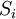$S_i$ indicates the state of the i-th site, and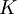$K$ is the coupling constant.

For a detailed and very readable history of the Lenz-Ising model see the following references:  .

## 1-dimensional Ising model

Main article: 1-dimensional Ising model

The 1-dimensional Ising model has an exact solution.

## 2-dimensional Ising model

The 2-dimensional square lattice Ising model was solved by Lars Onsager in 1944    after Rudolf Peierls had previously shown that, contrary to the one-dimensional case, the two-dimensional model must have a phase transition  .

#### Critical temperature

The critical temperature of the 2D Ising model is given by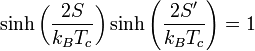$\sinh \left( \frac{2S}{k_BT_c} \right) \sinh \left( \frac{2S'}{k_BT_c} \right) =1$

where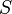$S$ is the interaction energy in the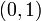$(0,1)$ direction, and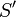$S'$ is the interaction energy in the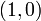$(1,0)$ direction. If these interaction energies are the same one has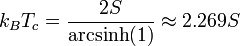$k_BT_c = \frac{2S}{ \operatorname{arcsinh}(1)} \approx 2.269 S$

#### Critical exponents

The critical exponents are as follows:

• Heat capacity exponent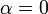$\alpha = 0$ (Baxter Eq. 7.12.12)
• Magnetic order parameter exponent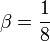$\beta = \frac{1}{8}$ (Baxter Eq. 7.12.14)
• Susceptibility exponent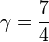$\gamma = \frac{7}{4}$ (Baxter Eq. 7.12.15)

## 3-dimensional Ising model

Sorin Istrail has shown that the solution of Ising's model cannot be extended into three dimensions for any lattice   In three dimensions, the critical exponents are not known exactly. However, Monte Carlo simulations, renormalisation group analysis and conformal bootstrap techniques provide accurate estimates :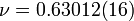$\nu=0.63012(16)$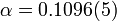$\alpha=0.1096(5)$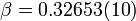$\beta= 0.32653(10)$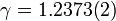$\gamma=1.2373(2)$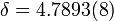$\delta=4.7893(8)$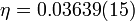$\eta =0.03639(15)$

with a critical temperature of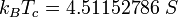$k_BT_c = 4.51152786~S$

## ANNNI model

The axial next-nearest neighbour Ising (ANNNI) model  is used to study spatially modulated structures in alloys, adsorbates, ferroelectrics, magnetic systems, and polytypes.

## Cellular automata

The Ising model can be studied using cellular automata .